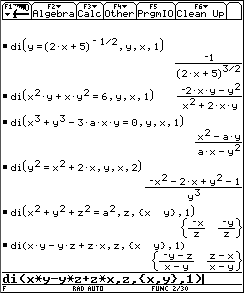## Texas Instruments TI-89 and TI-92 Plus Computer

Last page update: Sunday, November 21, 1999

 Multivariable Implicit Derivative - for the TI-89 and TI-92 Plus Version 1.0   (Monday, September 10, 1999) Download [ di.89f  | di.9xf ] (643 bytes) Description Determines single or multivariable implicit derivative to the first or greater order. Screen shotsDocumentation Usage:  di(Expr,ImplicitVar,ExplicitVarOrList,Order)  Expr can be a function, expression, or equation. If Expr is not an equation, it will be assumed to be equal to zero (0).  ImplicitVar is the implicit variable.  ExplicitVarOrList is the explicit variable or a list of one or more explicit variables in an expression of three of more variables.  Return value:  The implicit derivative or a list of implicit derivatives if ExplicitVarOrList is a list of two or more variables.  Examples:  1. di(y=(2x+5)-1/2,y,x,1)  (y is an implicit function of x, first order)      -(2x+5)-3/2  2. di(y2=x2+2x,y,x,2)  (y is an implicit function of x, second order)      (-x2-2x+y2-1)/y3 3. di(x2+y2+z2=a2,z,{x,y},1)  (z is an implicit function of x and y, first order)      (-x/z, -y/z}